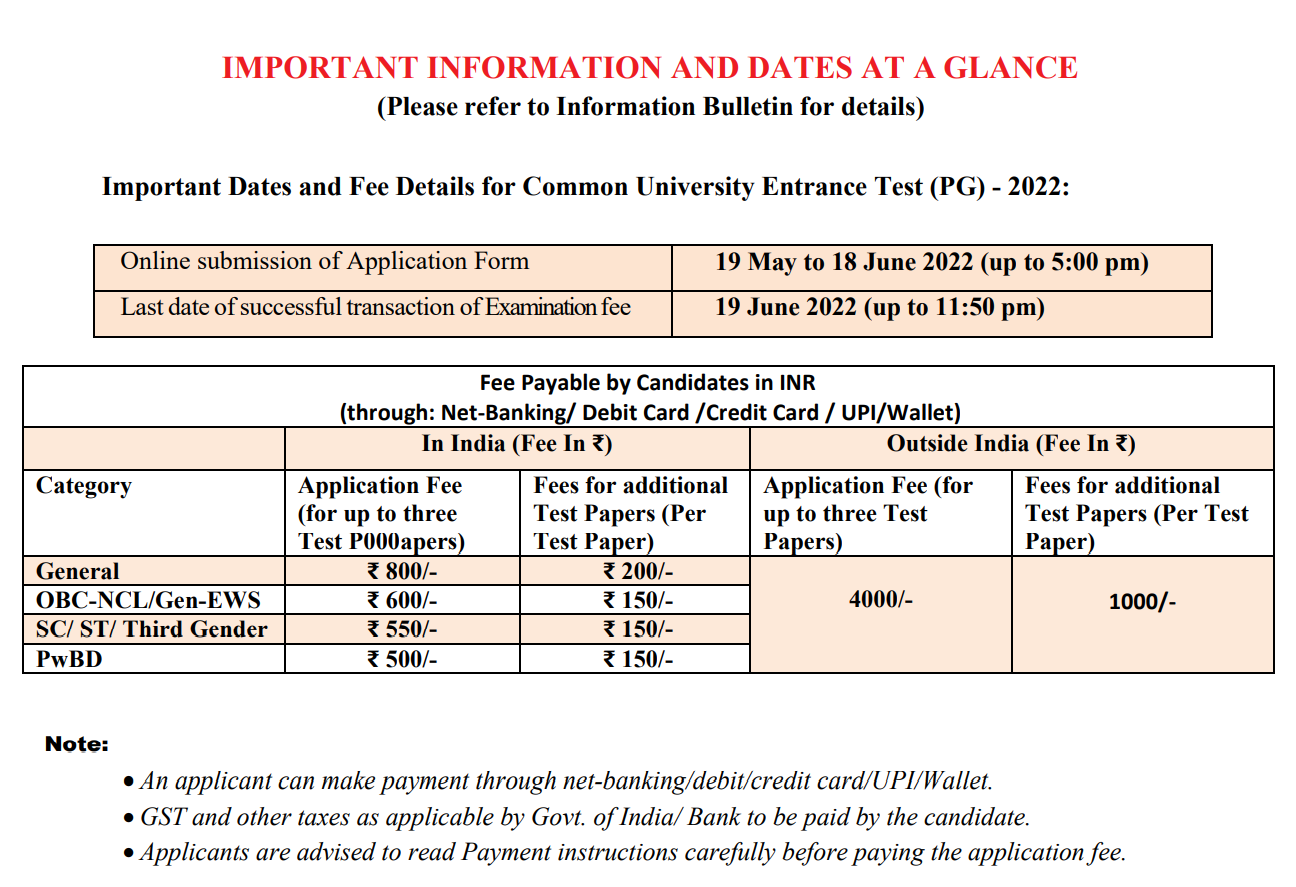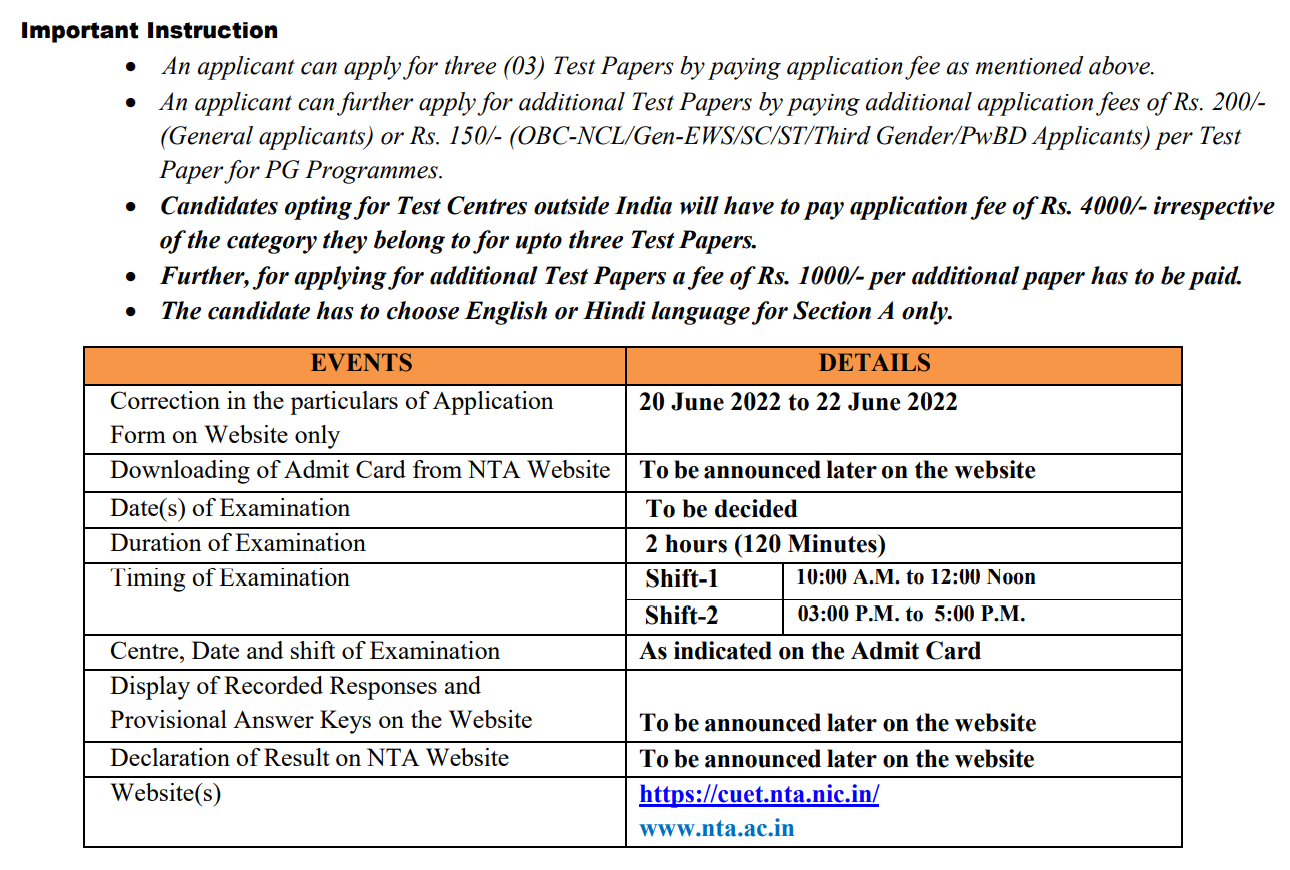# CUET – 2022 (Common University Entrance Test 2022)

CUET – 2022 (Common University Entrance Test 2022) Notification by Jitendra Mishra Academy (JMA), Indore
India’s No. 1 Institute for MCA Entrance Preparation…… Best MCA Entrance Coaching in India…. Top MCA Entrance Coaching in India….

Admission for Master of Computer Application (MCA) – 2022

Test date : To be decided

Eligibility : BHU MCA 2022

Obtained at least 60% marks in aggregate or 6.5 on a 10 -point scale (55% marks in aggregate or 6.0 on a 10-point scale in case of candidates belonging to reserved category) in
(i) B.C.A. / BIT /B.Tech. / BSc. With Computer Science as main or ancillary subject or equivalent Degree. or
(ii) B.Tech./B.E. in any branch (will be required to take bridge courses as per University rules)
or
(iii) B.A./ B.Sc. in Mathematics/ Statistics or equivalent Degree (will be required to take bridge courses as per University rules)
Pattern 1 (25 General + 75 Domain Specific Knowledge Questions)
Test Code : PGQP26

Eligibility : JNU MCA 2022

B.C.A./ Bachelor Degree in Computer Science Engineering or equivalent Degree with at least 55% marks OR B.Sc./ B.Com./ B.A. with Mathematics at 10+2 Level
or
at Graduation Level with at least 55% marks.
(All OBC category (non creamy layer) candidates are eligible to 10% relaxation in the percentage of marks in the qualifying examination in relation to open category. The SC/ST and Person with Disability (PWD) candidates who have passed the qualifying examination irrespective of their percentage of marks are eligible to appear in the Entrance Examination.)
Pattern 1 (25 General + 75 Domain Specific Knowledge Questions)
Test Code : PGQP26

Syllabus : CUET 2022 (Master of Computer Applications)

Thinking and Decision Making: Creative thinking, unfamiliar relationships, verbal reasoning, finding patterns trends and Assessment of figures & diagrams.

 Geometrical designs &Identification

 Selection of related letters / words / numbers /figures

 Identification of odd thing / item out from a group

 Completion of numerical series based on the pattern /logic

 Fill in the blanks of the series based on the numerical pattern and logic of the series

 Syllogisms (logic based questions), Identification of logic & selection of correct answers based on the logic Mathematics:

 Set Theory: Concept of sets – Union, Intersection, Cardinality, Elementary counting; permutations and combinations. • Probability and Statistics: Basic concepts of probability theory, Averages, Dependent and independent events, frequency distributions, measures of central tendencies and dispersions.

 Algebra: Fundamental operations in algebra, expansions, factorization, simultaneous linear /quadratic equations, indices, logarithms, arithmetic, geometric and harmonic progressions, determinants and matrices.

 Coordinate Geometry: Rectangular Cartesian coordinates, distance formulae, equation of a line, and intersection of lines, pair of straight lines, equations of a circle, parabola, ellipse and hyperbola.

 Calculus: Limit of functions, continuous function, differentiation of function, tangents and normals, simple examples of maxima and minima. Integration of functions by parts, by substitution and by partial fraction, definite integrals, applications of definite integrals to areas.

 Vectors: Position vector, addition and subtraction of vectors, scalar and vector products and their applications to simple geometrical problems and mechanics.

 Trigonometry: Simple identities, trigonometric equations, properties of triangles, solution of triangles, heights and distances, general solutions of trigonometric equations.

Computer Awareness:

 Computer Basics: Organization of a computer, Central Processing Unit (CPU), structure of instructions in CPU, input/output devices, computer memory, and back-up devices.

 Data Representation: Representation of characters, integers and fractions, binary and hexadecimal representations, binary arithmetic: addition, subtraction, multiplication, division, simple arithmetic and two’s complement arithmetic, floating point representation of numbers, Boolean algebra, truth tables, Venn diagrams.